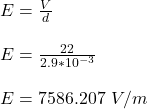An air-filled capacitor consists of two parallel plates, each with an area of 8 cm^2 , separated by a distance 2.9 mm. A 22 V potential diff

Question

An air-filled capacitor consists of two parallel plates, each with an area of 8 cm^2 , separated by a distance 2.9 mm. A 22 V potential difference is applied to these plates.
What is the magnitude of the surface charge density on each plate?

in progress 0
6 months 2021-08-04T07:11:48+00:00 1 Answers 2 views 0

The magnitude of the surface charge density on each plate is 6.714 x 10⁻ C/m²

Explanation:

Given;

area of the parallel plates, A = 8 cm² = 8 x 10⁻⁴ m²

distance between the plates, d = 2.9 mm = 0.0029 m

potential difference applied to the plates, V = 22 V

The electric field between the plates is given by;The surface charge density is given by;

σ = ε₀E

σ = (8.85 x 10⁻¹²)(7586.207)

σ = 6.714 x 10⁻ C/m²

Therefore, the magnitude of the surface charge density on each plate is 6.714 x 10⁻ C/m²